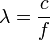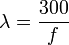# Wavelength

Wavelength is a property of an oscillation, vibration, or other regularly-repeating (cyclic) phenomenon. The wavelength of such a phenomenon is the reciprocal of an individual repetition of the repeating event. In the SI system of units, frequency is measured in Hertz (Hz), the number of repetitions (cycles) in one second.

Frequency ( f ) is inversely proportional to wavelength ( λ ), which is a length measurement:$\lambda = \frac{c}{f} \,\!$

For electromagnetic waves propagating in a vacuum, c = 299,792,458 m/s = 300 Mm/s (the speed of light). Rounding to convenient values for radio waves, 300 divided by the frequency in megahertz (Mhz) gives the wavelength in meters:$\lambda = \frac{300}{f} \,\!$

Three systems of designating ranges of frequencies and wavelengths are in common use:Some content on this page may previously have appeared on Citizendium.